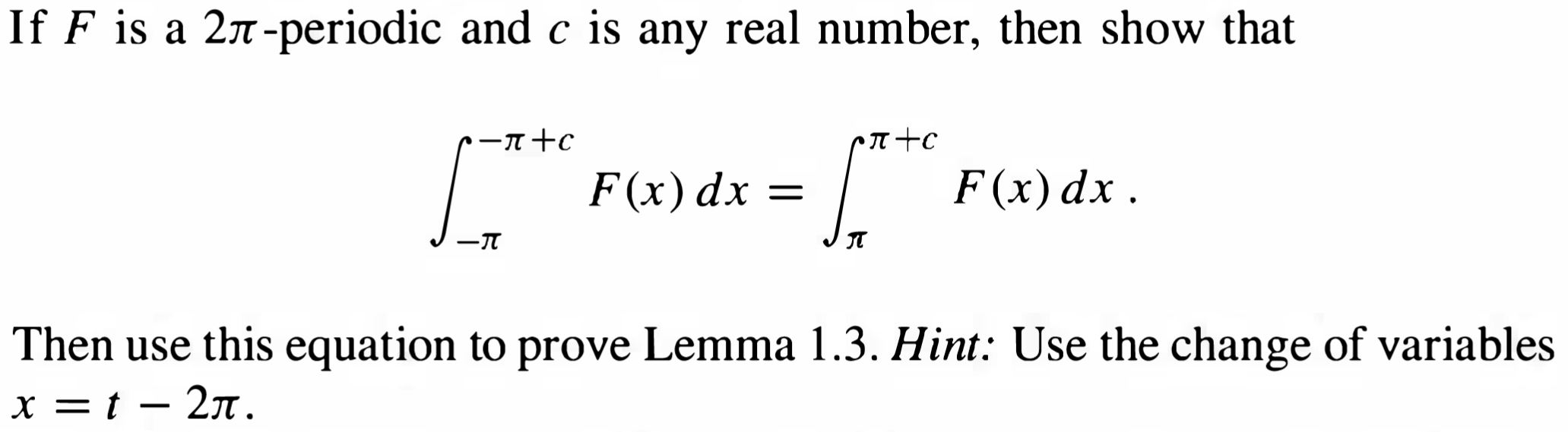Home / Answered Questions / Other / if-f-is-a-24-periodic-and-c-is-any-real-number-then-show-that-r-r-c-prto-i-f-x-dx-f-x-dx-j-n-then-us-aw591

# (Solved): If F Is A 24-periodic And C Is Any Real Number, Then Show That R-r+c Prto I F(x) Dx = F(x)dx. J -N T...If F is a 24-periodic and c is any real number, then show that r-r+c prto I F(x) dx = F(x)dx. J -N Then use this equation to prove Lemma 1.3. Hint: Use the change of variables x = t â€“ 21.

We have an Answer from Expert